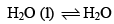Courses

# Which of the following is true for the reaction:at 1000C at one atmosphere:a)ΔE = 0                      b)ΔH = 0                  c)ΔH = ΔE              d)ΔH = TΔSCorrect answer is option 'D'. Can you explain this answer? Related Test: Test: Thermodynamics And Thermochemistry - 1

## Chemistry QuestionArup Guria Jul 22, 2018
As in equilibrium del G is zero from the following relation we get this answer del G = del H - T del SKrishna Kumar Aug 04, 2018
DG=0 for this process and as we know dG=dH-TdS therefore dH-TdS=0 hence dH=TdSRaji Nunna Oct 12, 2019
It is an isothermal process also so ∆E=0 ??G=H-TS at eqilibrium G is zero then H=TS in simply wordsAsf Institute Feb 26, 2021
H2​O(l) ​⇋ H2​O(g)​
As we know that, at equilibrium, ΔG=0
∴ΔH−TΔS=0
⇒ΔH=TΔSDhilip Kumar Aug 12, 2019
BRiu Sen Oct 19, 2019
DG =0 in equilibrium , we know that dG = dH -TdS
so , dH =TdS

This discussion on Which of the following is true for the reaction: at 1000C at one atmosphere:a)E = 0 b)H = 0 c)H = E d)H = TSCorrect answer is option 'D'. Can you explain this answer? is done on EduRev Study Group by Chemistry Students. The Questions and Answers of Which of the following is true for the reaction: at 1000C at one atmosphere:a)E = 0 b)H = 0 c)H = E d)H = TSCorrect answer is option 'D'. Can you explain this answer? are solved by group of students and teacher of Chemistry, which is also the largest student community of Chemistry. If the answer is not available please wait for a while and a community member will probably answer this soon. You can study other questions, MCQs, videos and tests for Chemistry on EduRev and even discuss your questions like Which of the following is true for the reaction: at 1000C at one atmosphere:a)E = 0 b)H = 0 c)H = E d)H = TSCorrect answer is option 'D'. Can you explain this answer? over here on EduRev! Apart from being the largest Chemistry community, EduRev has the largest solved Question bank for Chemistry.

### Related Content

This discussion on Which of the following is true for the reaction: at 1000C at one atmosphere:a)E = 0 b)H = 0 c)H = E d)H = TSCorrect answer is option 'D'. Can you explain this answer? is done on EduRev Study Group by Chemistry Students. The Questions and Answers of Which of the following is true for the reaction: at 1000C at one atmosphere:a)E = 0 b)H = 0 c)H = E d)H = TSCorrect answer is option 'D'. Can you explain this answer? are solved by group of students and teacher of Chemistry, which is also the largest student community of Chemistry. If the answer is not available please wait for a while and a community member will probably answer this soon. You can study other questions, MCQs, videos and tests for Chemistry on EduRev and even discuss your questions like Which of the following is true for the reaction: at 1000C at one atmosphere:a)E = 0 b)H = 0 c)H = E d)H = TSCorrect answer is option 'D'. Can you explain this answer? over here on EduRev! Apart from being the largest Chemistry community, EduRev has the largest solved Question bank for Chemistry.